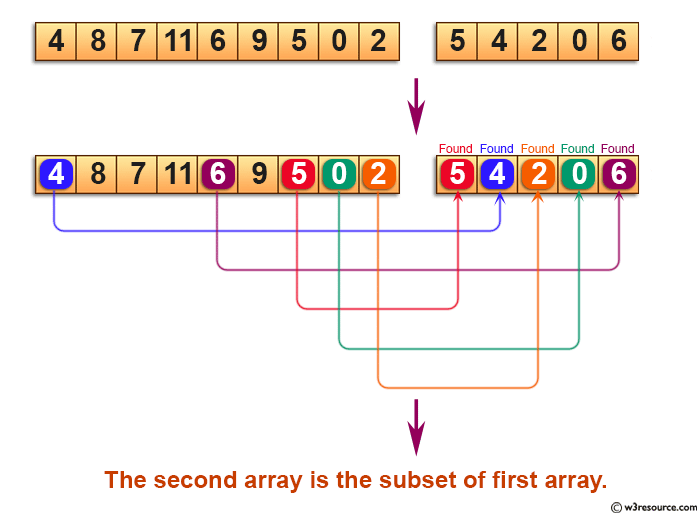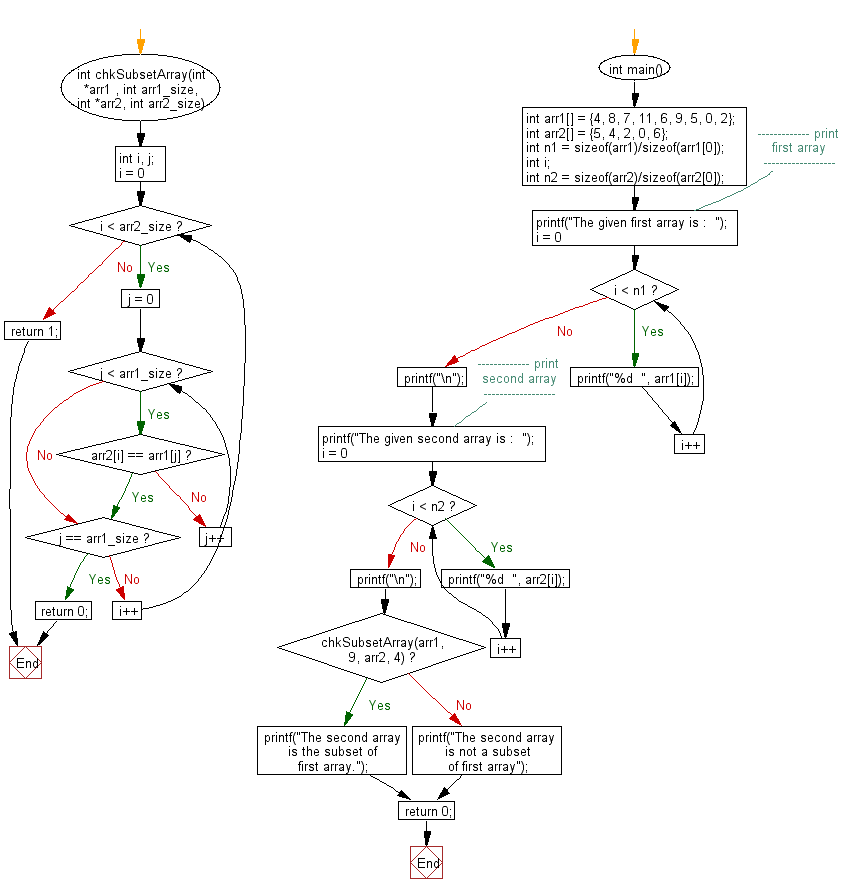﻿ C exercises: Check whether an array is subset of another array - w3resource# C Exercises: Check whether an array is subset of another array

## C Array: Exercise-55 with Solution

Write a program in C to check whether an array is subset of another array.

Pictorial Presentation:Sample Solution:

C Code:

``````#include <stdio.h>
int chkSubsetArray(int *arr1 , int arr1_size, int *arr2, int arr2_size)
{
int i, j;
for (i = 0; i < arr2_size; i++)
{
for (j = 0; j < arr1_size; j++)
{
if(arr2[i] == arr1[j])
break;
}
if(j == arr1_size)
return 0;
}
return 1;
}
int main()
{
int arr1[] = {4, 8, 7, 11, 6, 9, 5, 0, 2};
int arr2[] = {5, 4, 2, 0, 6};
int n1 = sizeof(arr1)/sizeof(arr1);
int i;
int n2 = sizeof(arr2)/sizeof(arr2);
//------------- print first array ------------------
printf("The given first array is :  ");
for(i = 0; i < n1; i++)
{
printf("%d  ", arr1[i]);
}
printf("\n");
//------------- print second array ------------------
printf("The given second array is :  ");
for(i = 0; i < n2; i++)
{
printf("%d  ", arr2[i]);
}
printf("\n");
//------------------------------------------------------
if(chkSubsetArray(arr1, 9, arr2, 4))
printf("The second array is the subset of first array.");
else
printf("The second array is not a subset of first array");

return 0;
}```
```

Sample Output:

```The given first array is :  4  8  7  11  6  9  5  0  2
The given second array is :  5  4  2  0  6
The second array is the subset of first array.
```

Flowchart:C Programming Code Editor:

Improve this sample solution and post your code through Disqus.

What is the difficulty level of this exercise?

﻿

## C Programming: Tips of the Day

Where is the C auto keyword used?

auto is a modifier like static. It defines the storage class of a variable. However, since the default for local variables is auto, you don't normally need to manually specify it.

Ref : https://bit.ly/3yzwC9r